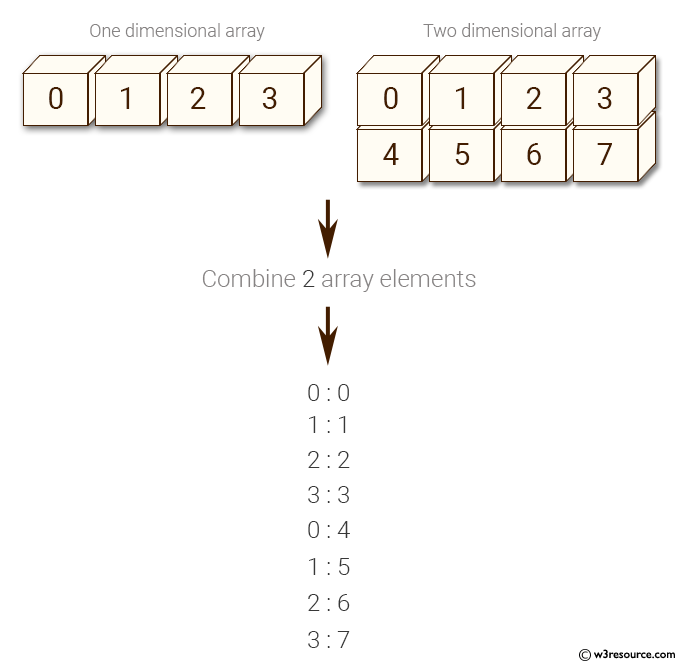﻿ NumPy: Combine a one and a two dimensional array together and display their elements - w3resource# NumPy: Combine a one and a two dimensional array together and display their elements

## NumPy: Array Object Exercise-74 with Solution

Write a NumPy program to combine a one and a two dimensional array together and display their elements.

Pictorial Presentation:Sample Solution:-

Python Code:

``````import numpy as np
x = np.arange(4)
print("One dimensional array:")
print(x)
y = np.arange(8).reshape(2,4)
print("Two dimensional array:")
print(y)
for a, b in np.nditer([x,y]):
print("%d:%d" % (a,b),)
```
```

Sample Output:

```One dimensional array:
[0 1 2 3]
Two dimensional array:
[[0 1 2 3]
[4 5 6 7]]
0:0
1:1
2:2
3:3
0:4
1:5
2:6
3:7
```

Python Code Editor:

Have another way to solve this solution? Contribute your code (and comments) through Disqus.

What is the difficulty level of this exercise?

Test your Python skills with w3resource's quiz

﻿

## Python: Tips of the Day

Set comprehension:

```>>> m = {x ** 2 for x in range(5)}
>>> m
{0, 1, 4, 9, 16}
```